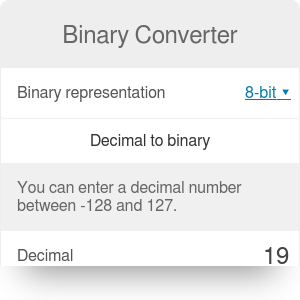Decimal to binary
Decimal number
Binary number
Binary to decimal
Binary number
Decimal number

# Binary Converter

By Bogna Haponiuk

The binary converter is a handy tool that will enable you to quickly perform a conversion of numbers. You will be able to use it both as a binary to decimal converter and as a decimal to binary calculator. Read on to learn what is the binary system, how to convert the numbers and how to use this calculator to obtain correct results.

## What is the binary system?

We normally operate in a decimal system. That means that we use ten different digits, from 0 to 9. If you write down a number, for example 345, each consecutive digit of this number corresponds to a different power of ten. That means that:

• Digit 5 corresponds to 10⁰ (5 is equal to `5 * 10⁰`).
• Digit 4 corresponds to 10¹ (40 is equal to `4 * 10¹`).
• Digit 3 corresponds to 10² (300 is equal to `3 * 10²`).

In the binary system, there are only two available digits: 0 and 1. That means that the digits of every number, instead of corresponding to powers of 10, correspond to powers of 2. For example, the number 1111 in binary system can be analyzed in the following way:

• The last 1 corresponds to 2⁰ (1 is equal to `1 * 2⁰ = 1`).
• The second-to-last 1 corresponds to 2¹ (10 is equal to `1 * 2¹ = 2`).
• Third-to-last 1 corresponds to 2² (100 is equal to `1 * 2² = 4`).
• The first 1 corresponds to 2³ (1000 is equal to `1 * 2³ = 8`).

After adding all of these numbers, we get `1 + 2 + 4 + 8 = 15`. Number 1111 in the binary system corresponds to 15 in the decimal system.

## Converting from decimal to binary

You can use a very simple and efficient algorithm to convert numbers from the decimal to binary system.

1. Take your initial number. Divide it by 2.
2. Note down the remainder. It is going to be equal to 0 or 1. This will be the last digit of the binary number (the rightmost one).
3. Take the quotient. It is your new "initial number".
4. Keep repeating the above steps, each time adding the remainder to the left of previously obtained digits.

For example, for number 19, we would have the following steps:

1. 19/2 = 9, remainder 1
2. 9/2 = 4, remainder 1
3. 4/2 = 2, remainder 0
4. 2/2 = 1, remainder 0
5. 1/2 = 0, remainder 1

Reading from the bottom to the top, 19 corresponds to 10011 in binary system. Check this result with the binary converter!

## Converting from binary to decimal

It is similarly easy - all you have to do is reverse the algorithm explained above:

1. Take the leftmost digit of your initial number. Multiply it by 2.
2. Add the next digit of the binary number. The sum will be your new "initial number".
3. Keep repeating these steps, each time first multiplying by 2 and then adding the last digit.

For example, for the binary number 110011, we would have the following steps:

1. `1 * 2 = 2`
2. `(2 + 1) * 2 = 6`
3. `(6 + 0) * 2 = 12`
4. `(12 + 0) * 2 = 24`
5. `(24 + 1) * 2 = 50`
6. `50 + 1 = 51`

110011 corresponds to 51 in decimal system. Check this result with the binary converter!

Bogna Haponiuk

## Get the widget!

Binary Converter can be embedded on your website to enrich the content you wrote and make it easier for your visitors to understand your message.

It is free, awesome and will keep people coming back!